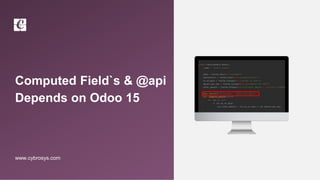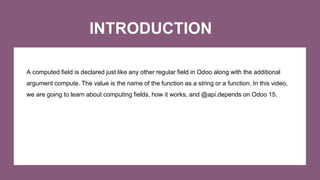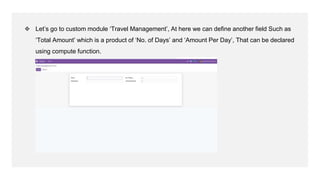Se está descargando tu SlideShare. ×

# What is Computed Fields and @api Depends in Odoo 15

Anuncio
Anuncio
Anuncio
Anuncio
Anuncio
Anuncio
Anuncio
Anuncio
Anuncio
Anuncio
AnuncioCargando en…3
×

1 de 16 Anuncio

# What is Computed Fields and @api Depends in Odoo 15

This slide discusses compute function and how to store value in a field using this functionality @api.depends operation. The value is the name of the function.

This slide discusses compute function and how to store value in a field using this functionality @api.depends operation. The value is the name of the function.

Anuncio
Anuncio

### What is Computed Fields and @api Depends in Odoo 15

1. 1. Computed Field`s & @api Depends on Odoo 15 www.cybrosys.com
2. 2. INTRODUCTION A computed field is declared just like any other regular field in Odoo along with the additional argument compute. The value is the name of the function as a string or a function. In this video, we are going to learn about computing fields, how it works, and @api.depends on Odoo 15.
3. 3. ❖ Let’s go to custom module ‘Travel Management’, At here we can define another field Such as ‘Total Amount’ which is a product of ‘No. of Days’ and ‘Amount Per Day’, That can be declared using compute function.
4. 4. ❖ So, at the pycharm, Let’s define that field, here i am defining it as ‘Total Amount’ as an ‘Integer’ field. First step in the compute function is that, we need to define that compute function along with the field. That is, ‘compute=’_compute_amount’’. Here The ‘_compute_amount’ is the function name.
5. 5. ❖ Then we have to return the function, So first thing we need to add is ‘@api.depends’, for that we need to import ‘api’ from odoo, ‘@api.depends’ is an ‘api’ operation it depends on some other field of same table or some other table. Which means our ‘total_amount’ is depend upon the ‘no_of_days’ and ‘amount_per_day’. So in the ‘@api.depends’ operation we can add those two fields.
6. 6. ❖ Here one of the field get changed it will depend upon the ‘total_amount’ that we are going to compute.And let’s define our compute function like below, ❖ So, This function_name we declared in the field ‘total_amount’.
7. 7. ❖ Then we can loop through each records.
8. 8. ❖ Here add a loop for this function. That is, if ‘no_of_days’ is available.
9. 9. ❖ Then Let’s define the amount. That is, the product of ‘rec.no_of_days’ and ‘rec.amount_per_day’ is stored in the field ‘rec.total_amount’.
10. 10. ❖ Instead of the else condition setting it as ‘rec.total_amount’ is false. This means if there is no ‘no_of_days’ is given.
11. 11. ❖ Now, We have to add the ‘total_amount’ field in the xml also,
12. 12. ❖ After Upgrading the module, Let’s check the field in the ‘travel.travel’ model. Here you can see the Field ‘total_amount’
13. 13. ❖ Here, Let’s see the field ‘total_amount’, which is the product of ‘no_of_days’ and ‘amount_per_day’. Likewise The compute function works.
14. 14. For More Details Check our company website for related blogs and Odoo book. Odoo Book V15 Check our YouTube channel for functional and technical videos in Odoo. Computed Fields and @api Depends on Odoo 15
15. 15. Thank You